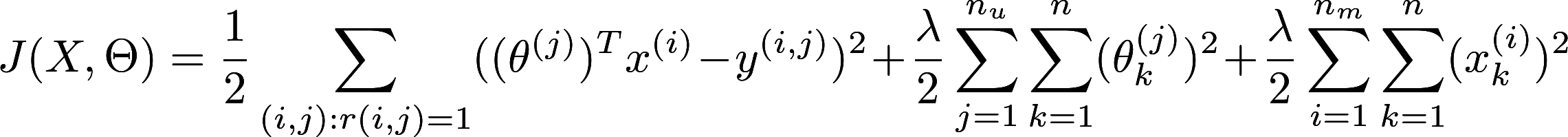How can you display mathematical equation in HTML? MathJax can help.
Post
Cancel

# How can you display mathematical equation in HTML? MathJax can help.Recently I need to joint notes during my machine learning beginner course offered by Andrew Ng. I would like to share them in the following few weeks. So I shopped for some solutions for me to put a lot of mathematical equations, formatted beautifully, in here. I spent 5 minutes on searching, realizing the solution is right in front of me. I am taking the course from Coursera, and Coursera used MathJax as their solution. This solved my problem brilliantly as it is very comprehensive in displaying mathematical equations.

What is so brilliant of MathJax? MathJax is able to display LATEX in HTML page, here is an example:

$J(\theta) =\frac{1}{2m}[\sum^m_{i=1}(h_\theta(x^{(i)}) - y^{(i)})2 + \lambda\sum^n_{j=1}\theta^2_j$

All the LATEX code is as follows.

1 2 3 J(\theta) =\frac{1}{2m} [\sum^m_{i=1}(h_\theta(x^{(i)}) - y^{(i)})2 + \lambda\sum^n_{j=1}\theta^2_j 

Not only from LATEX to HTML, MathJax translates different usual mathematical notation markup languages into different web format. For example you could input MathML and MathJAX could output a SVG representation. So if you are familiar with any of these language, you can put it on the web.

### But I am not a tech person, I don’t know much LATEX…

Equation Editor could help you. It is an visual editor for complex mathematical equation. Not only the result, you can see also the LATEX code too. Also LATEX is super useful if you always need to make complex documents, consider learning it with for free online.

### I am sold, how to install it?

It is very easy. In the HTML scripts block, you first need to add MathJax as one of your javascript code.

1 2 3 4 <script type="text/javascript" async src="https://cdn.mathjax.org/mathjax/latest/MathJax.js/MathJax.js?config=TeX-MML-AM_CHTML"> </script> 

Bear in mind that there is different default config, you could check out the config documentation

And inside the document, whether it is markdown or HTML, you could use delimiter to signal the start and end of the equations.So you could enclose your LATEX code inside two delimiters. The default delimiter is $$. Here is an example for the delimiter. 1$$ {your LATEX code}